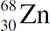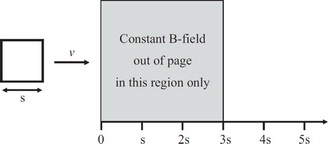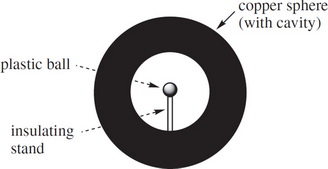# AP Physics 2 Practice Test 4

### Test Information10 questions18 minutes

1. What happens to the pressure, P, of an ideal gas if the temperature is increased by a factor of 2 and the volume is increased by a factor of 8 ?

2. Two nuclides that have the same excess number of neutrons over protons are called isodiaphers. Which of the following is an isodiaphere of?

3. The plates of a capacitor are charged to a potential difference of 5 V. If the capacitance is 2 mF, what is the charge on the positive plate?

4. A confined ideal gas undergoes a cyclical process in three steps—an isobaric step, followed by an isochoric step, followed by an isothermal step. Which of the following must be true?

5. A liquid flows at a constant flow rate through a pipe with circular cross-sections of varying diameters. At one point in the pipe, the diameter is 2 cm and the flow speed is 18 m/s. What is the flow speed at another point in this pipe, where the diameter is 3 cm?

6. A bi-convex lens has a radius of curvature of magnitude 20 cm. Which of the following best describes the image formed of an object of height 2 cm placed 30 cm from the lens?

7.A square loop of conducting wire with side s is moved at a constant rate V to the right into a region where there is a constant magnetic field directed out of the page. Which of the following graphs shows the flux through the loop as a function of distance?

8.The figure above shows a solid copper sphere carrying a charge of +1 mC that contains a hollow cavity. Which of the following best describes what will occur when a plastic ball carrying a charge of +1 mC is placed inside the cavity?

9. Wire Y is made of the same material but has twice the diameter and half the length of Wire X. If Wire X has a resistance of Q then Wire Y would have a resistance of

10. How much work is required to charge a 10 µF capacitor to a potential difference of 100 V ?